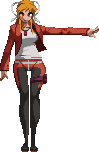# Triggers - Trigger Logic (CNS) (Read 4146 times)

Started by Just No Point, October 06, 2015, 10:41:46 pm

#### Just No Point##### Triggers - Trigger Logic (CNS)
#1  October 06, 2015, 10:41:46 pm
••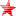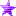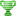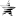The first trigger should always be trigger1, and subsequent triggers should be trigger2, then trigger3 and so on. The logic for deciding if a controller should be activated is:

Are all conditions of trigger1 true? If so, then yes, activate the controller.
Otherwise, repeat the test for trigger2, and so on, until no more triggers are found.
This can be thought of as "OR" logic.

Be careful; skipping numbers will cause some triggers to be ignored. For example, if you have triggers trigger1, trigger2 and trigger4 without a trigger3, then trigger4 will be ignored.

Now what if you want more than one condition to be met before the controller is activated? Here is an commonly-used example for testing if a player in the air has reached the ground. The triggers used are:

Code:
`trigger1 = Vel Y > 0 ; True if Y-velocity is > 0 (going down)trigger1 = Pos Y > 0 ; True if Y-position is > 0 (below ground)`
At this point, you may be confused by the format of the trigger. Do not worry about it for now. We will get to it soon.

As you can see above, both the triggers have the same number. When several triggers have the same number, it implements "AND" logic. That is, the controller is activated if every one of the triggers with the same number is true, but not if one or more of them is false.

You can combine both ideas. For example:

Code:
`trigger1 = Vel Y > 0 ; True if Y-velocity is > 0 (going down)trigger1 = Pos Y > 0 ; True if Y-position is > 0 (below ground)trigger2 = Time = 5  ; True if state-time is 5`
The controller for this would be activated if the player landed on the ground (y-velocity and y-Position are both > 0), OR if his state time was 5.

Here is a summary:

Triggers with the same number activate the controller only if all of them are true.
Triggers with different numbers activate the controller if any one or more of them are true.
The format of a trigger is:

trigger? = condition_exp
condition_exp is an arithmetic expression to be checked for equality to 0. If condition_exp is 0, then the trigger is false. If condition_exp is nonzero, then the trigger is true. The condition_exp is usually a simple relational expression as in the examples above, but can be as simple or as complicated as required.

It is possible to use logical operators between expressions. For instance, this is equivalent to the previous example above.

trigger1 = ((Vel Y > 0) && (Pos Y > 0)) || Time = 5
See Expressions for a detailed explanation of arithmetic expressions.

A useful shortcut you might use is triggerall. It specifies a condition that must be true for all triggers. For instance, consider:

Code:
`triggerall = Vel X = 0trigger1 = Pos Y > -2trigger2 = AnimElem = 3trigger3 = Time = [2,9]`
For any of trigger1 to trigger3 to be checked, the triggerall condition must be true too. In this case, as long as the x-velocity is not 0, then the state controller will not be activated. You can have more than one triggerall condition if you need. Note that at least one trigger1 must be present, even if you specify triggerall.Last Edit: October 07, 2015, 03:44:38 am by Just No Point

#### Cyanide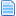##### Re: Triggers - Trigger Logic (CNS)
#2  October 07, 2015, 09:25:01 am
••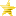• Legendary XIII
• I am the eye of the storm to come!
Although the above makes sense, many coders don't bother with the ordering. Aside from an increase in overall processing, this makes no actual difference. You could for example have

Code:
`trigger2 = Pos Y > -2    trigger3 = AnimElem = 3    triggerall = Vel X = 0    trigger1 = Time = [2,9]`
It'll still work, it's just harder to read. If you see this, there is nothing strictly wrong with it, as in, if you are looking for a fault, this will not be the cause. However it's not particularly good practice within how mugen works so avoid doing it.

Another gotcha within triggering that might catch you out is the following

trigger1 = time = [5,10] && animelemtime(3) > 2
This will break and cause mugen to quit
trigger1 = (time = [5,10]) && animelemtime(3) > 2
This will not
trigger1 = animelemtime(3) > 2 && time = [5,10]
Nor will this

Mugen is stupid sometimes.In M.U.G.E.N there is no magic button

They say a little knowledge is a dangerous thing, but it's not one half so bad as a lot of ignorance.

#### Odb718##### Re: Triggers - Trigger Logic (CNS)
#3  October 07, 2015, 01:54:20 pm
••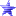• Shame on you!
You can combine both ideas. For example:

Code:
`trigger1 = Vel Y > 0 ; True if Y-velocity is > 0 (going down)trigger1 = Pos Y > 0 ; True if Y-position is > 0 (below ground)trigger2 = Time = 5  ; True if state-time is 5`
The controller for this would be activated if the player landed on the ground (y-velocity and y-Position are both > 0), OR if his state time was 5.

Depending on how it's actually played out in mugen, what ever you're triggering may happen twice. The OR part isnt entirely true. I'd say it's more like a, "also if". Because it'll Also happen If trigger2 is correct.
Take,

[State 0, Explod]
type = Explod
trigger1 = animelem = 4
trigger2 = animelem = 26

The explod would activate on both animelem 4 and 26.  You couldnt use
trigger1 = animelem = 4 && animelem = 26
because that's an impossibility. Though
trigger1 = animelem = 4 || animelem = 26
would work like normal. (and a little bit faster)

In order for your example to really be an OR situation it would read like
trigger1 = Vel Y > 0 && Pos Y > 0 ; True if Y-velocity is > 0 (going down) && if Y-position is > 0 (below ground)
trigger2 = Vel Y <= 0 && Pos Y <= 0 ;wont be true if trigger1 is true
trigger2 = Time = 5  ; True if state-time is 5

#### Zzyzzyxx##### Re: Triggers - Trigger Logic (CNS)
#4  April 30, 2016, 03:45:51 pm
••• I hate coding helpers.

Let's see this code:

trigger1 = vel y > 0
trigger3 = pos y > 0
trigger4 = movehit && numtarget
trigger5 = moveguarded
trigger6 = !animtime

In this code, the trigger2 doesn't exist. In this case, Mugen simply ignores the triggers 3, 4, 5 and 6. You won't see the char executing the action intended by you, the programmer, even if your char satisfies one of the conditions ignored due to the flawed code.

Although it sounds obvious, keep in mind that if the char fails to obey your code, this is one of the possible reasons. Just use the correct numerical order to fix this issue.

trigger1 = vel y > 0
trigger2 = pos y > 0
trigger3 = movehit && numtarget
trigger4 = moveguarded
trigger5 = !animtime